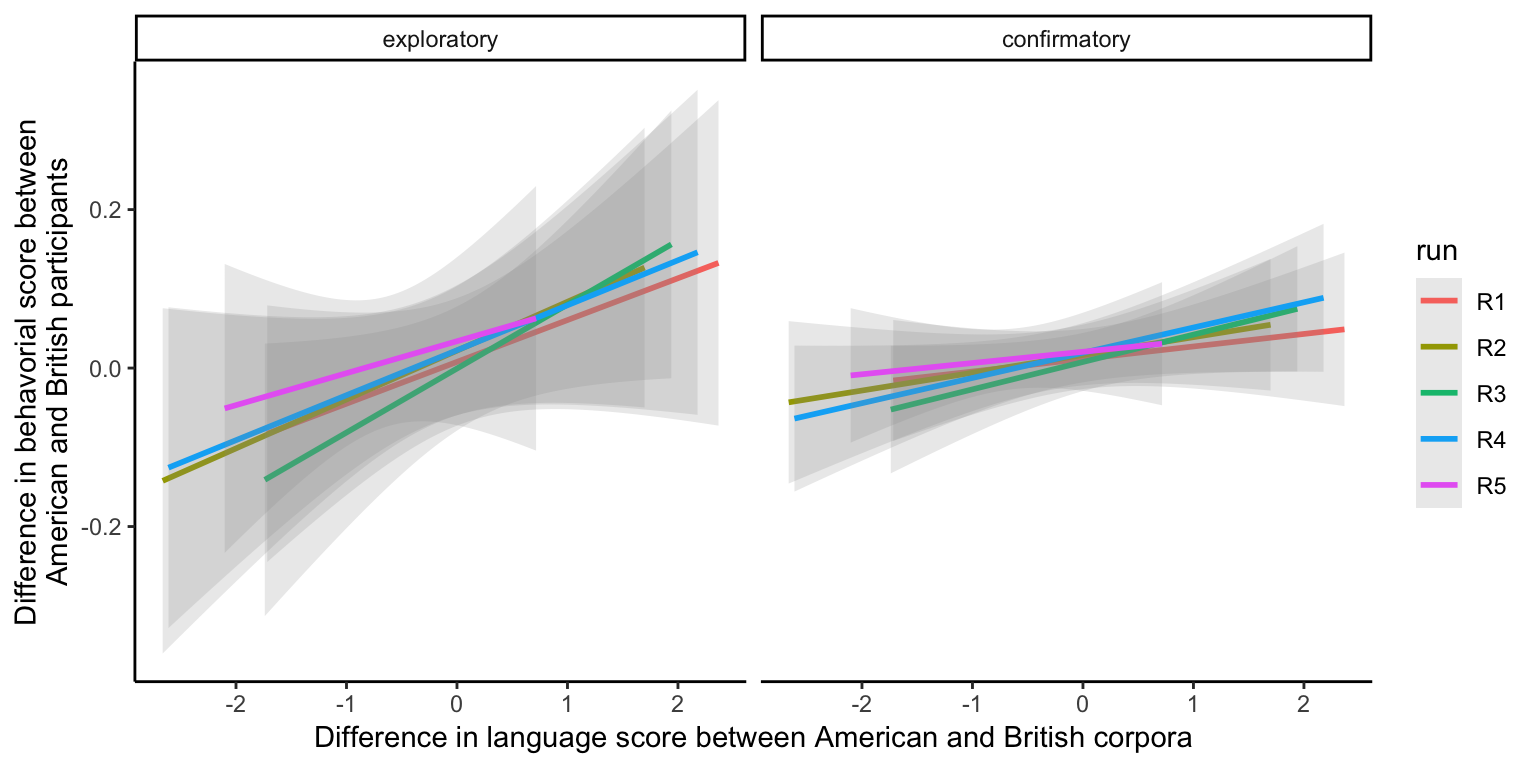This is a pre-registered study. The pre-registeration can be found here: https://osf.io/3f9ed/.

In this study, we’re interested in whether psychological biases in language are reflected in behavioral measures of bias, as measured by the implicit association task. This is a confirmatory analysis. To do this, we’re using an existing dataset (AIID; https://osf.io/pcjwf/) to compare performance on a range of different IATs (N = 31) between British vs. American English participants. We compared the difference in magnitude of these biases between British and American participants to differences in the magnitude of these biases in language. To measure the bias in language, we trained word embedding models on two corpora (BNC and COCA) and calculated the effect size of the bias in each, using the method described in Caliskan, et al. (2017). Our main hypothesis is that the difference in behavioral bias will be positively correlated with the difference in the language bias.

The raw exploratory IAT data was processed using the script found in `analyses/study1c/09_tidy_behav_IAT_data.R`. The language effect size estimates were produced from scripts in `analyses/study1c/`.

## Data

### Language effect size

FILENAME: `bnc_vs_coca_es_400_10_x5.csv`

We have estimates of the language IAT bias for each IAT (N = 31) from 5 different training runs of each of the two corpora (BNC and COCA).

``````LANGUAGE_PATH <- here("data/study1c/processed/bnc_vs_coca_es_400_10_x5.csv")

# language es (5 runs of each model)
es_lang_tidy <- es_lang_raw %>%
rename(coca_lang_es = coca,
bnc_lang_es = bnc) %>%
mutate(lang_diff = bnc_lang_es - coca_lang_es) # get bnc - coca language es difference

glimpse(es_lang_tidy)``````
``````## Observations: 155
## Variables: 5
## \$ run          <chr> "R1", "R1", "R1", "R1", "R1", "R1", "R1", "R1", "R1…
## \$ domain       <chr> "Athletic People - Intelligent People", "Avoiding -…
## \$ bnc_lang_es  <dbl> 0.38273260, -0.85778725, -0.96763137, 1.49612818, -…
## \$ coca_lang_es <dbl> 0.43262773, 0.85917319, 0.07219491, -0.87062804, 0.…
## \$ lang_diff    <dbl> -0.04989513, -1.71696043, -1.03982628, 2.36675622, …``````

Description of variables:

• `run` - Run ID of model (1-5)

• `domain` - IAT domain

• `bnc_lang_es` - Estimate of effect size in language based on model trained on BNC.

• `coca_lang_es` - - Estimate of effect size in language based on model trained on COCA

• `lang_diff` - Difference between BNC and COCA estimates.

### Behavioral effect size

FILENAME: `tidy_behavioral_iat_data.csv`

We have estimates of behavioral bias for each IAT for each sample of participants (UK and USA).

``````BEHAVIORAL_PATH <- here("data/study1c/processed/tidy_behavioral_iat_data_confirmatory.csv")
es_behavior_tidy_conf <- es_behavioral_raw_conf %>%
rename(us_behavior_es = us,
uk_behavior_es = uk) %>%
mutate(data_type = "confirmatory")

BEHAVIORAL_PATH_EXP <- here("data/study1c/processed/tidy_behavioral_iat_data.csv")
es_behavior_tidy_exp <- es_behavioral_raw_exp %>%
rename(us_behavior_es = us,
uk_behavior_es = uk) %>%
mutate(data_type = "exploratory")

es_behavior_tidy <- bind_rows(es_behavior_tidy_conf, es_behavior_tidy_exp)

glimpse(es_behavior_tidy)``````
``````## Observations: 62
## Variables: 5
## \$ domain                <chr> "Athletic People - Intelligent People", "A…
## \$ uk_behavior_es        <dbl> 0.08186083, 0.43526553, 0.19073844, 0.3408…
## \$ us_behavior_es        <dbl> -5.760283e-03, 4.595948e-01, 1.798045e-01,…
## \$ behavioral_resid_diff <dbl> 0.087621110, -0.024329291, 0.010933918, 0.…
## \$ data_type             <chr> "confirmatory", "confirmatory", "confirmat…``````

Description of variables:

• `domain` - IAT domain

• `uk_behavior_es` - Estimate of behavioral IAT effect size of UK participants.

• `us_behavior_es` - Estimate of behavioral IAT effect size of USA participants.

• `behavioral_resid_diff` - Difference between UK and USA estimates.

• `data_type` - Data from exploratory or confirmatory dataset.

``````#Join everything together.
tidy_iat_data <- es_lang_tidy %>%
full_join(es_behavior_tidy)  %>%
mutate(data_type = fct_rev(data_type))``````

## Models

### Difference correlations

Difference in behavioral scores as a function of difference in language scores. Each color corresponds to a different run of the model. Each point corresponds to a particular IAT (e.g. “Career - Family”).

``````### All IATs
## simple correlations
get_correlations <- function(current_df){
cor1 <- tidy(cor.test(current_df\$coca_lang_es, current_df\$us_behavior_es)) %>%
mutate(corr = "coca_us")
cor2 <- tidy(cor.test(current_df\$coca_lang_es, current_df\$uk_behavior_es)) %>%
mutate(corr = "coca_uk")
cor3 <- tidy(cor.test(current_df\$bnc_lang_es, current_df\$us_behavior_es)) %>%
mutate(corr =  "bnc_us")
cor4 <- tidy(cor.test(current_df\$bnc_lang_es, current_df\$uk_behavior_es)) %>%
mutate(corr = "bnc_uk")
cor5 <- tidy(cor.test(current_df\$bnc_lang_es, current_df\$coca_lang_es)) %>%
mutate(corr = "coca_bnc_corr")
cor6 <- tidy(cor.test(current_df\$uk_behavior_es, current_df\$us_behavior_es)) %>%
mutate(corr = "uk_us_corr")
cor7 <- tidy(cor.test(current_df\$behavioral_resid_diff, current_df\$lang_diff)) %>%
mutate(corr = "diff_corr")

bind_rows(list(cor1, cor2, cor3, cor4, cor5, cor6, cor7)) %>%
select(corr, estimate, statistic, p.value,
parameter, conf.low, conf.high) %>%
list()
}

# get correlation for each run
all_corrs <- tidy_iat_data %>%
group_by(run, data_type) %>%
nest() %>%
mutate(model = map(data, ~ get_correlations(.))) %>%
select(-data) %>%
unnest() %>%
unnest()

ggplot(tidy_iat_data, aes(x = lang_diff, y = behavioral_resid_diff,
color = run, group = run)) +
# ggrepel::geom_text_repel(aes(label = domain)) +
facet_grid(~ data_type) +
geom_smooth(method = "lm", alpha = .2) +
ylab("Difference in behavorial score between\nAmerican and British participants") +
xlab("Difference in language score between American and British corpora") +
theme_classic()````````````tidy_iat_data %>%
group_by(domain, data_type) %>%
summarize_if(is_numeric, mean)  %>%
ggplot(aes(x = lang_diff, y = behavioral_resid_diff,group = data_type)) +
geom_smooth(method = "lm", alpha = .2) +
geom_point(size = .6) +
ggrepel::geom_text_repel(aes(label = domain), size = 1.7) +
facet_grid(~ data_type) +
ylab("Difference in behavorial score between\nAmerican and British participants") +
xlab("Difference in language score between American and British corpora") +
theme_classic() +
theme(legend.position = "none")``````